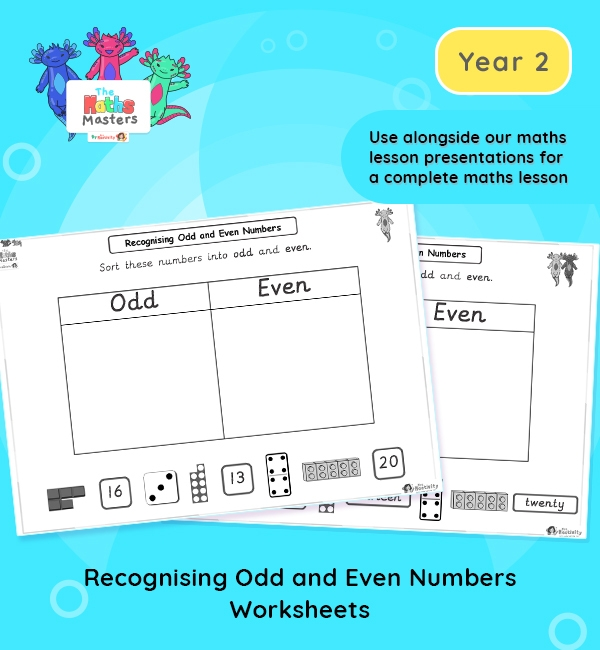# Year 2 | Recognising Odd and Even Numbers Worksheets## Year 2 odd and even worksheets

Aligned with the maths mastery approach, these differentiated Year 2 | Recognising Odd and Even Numbers Worksheets are designed to be used alongside our Year 2 | Odd and Even Numbers Lesson Presentation for a complete maths lesson. The maths worksheets require children to cut out numbers and place them in the odd or even section of the table.

Topic: Multiplication and division

Small Steps: Odd and even numbers.

NC Links: Recall and use multiplication and division facts for the 2, 5, and 10 times tables including recognising odd and even numbers. *Solve problems involving multiplication and division using materials, arrays, repeated addition, mental methods and multiplication and division facts including problems in context. *Count in steps of 2, 3, 5, and 10.

TAF Statements: Working At  – Recall and use multiplication and division facts for 2, 5, and 10 and use them to solve simple problems.

Greater Depth – Use reasoning about number and relationships to solve more complex problems and explain their thinking.

Ready-to-progress criteria:  2MD-1 Recognise repeated addition contexts, representing them with multiplication equations and calculating the product within the 2, 5, and 10 multiplication tables.

Year 1 conceptual prerequisites: Count in multiples of 2, 5 and 10

## Recently Viewed